Publicité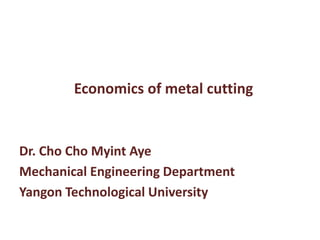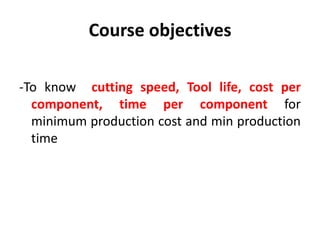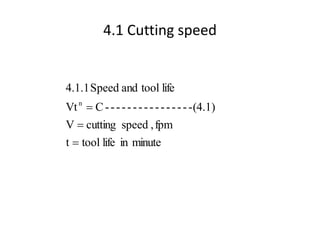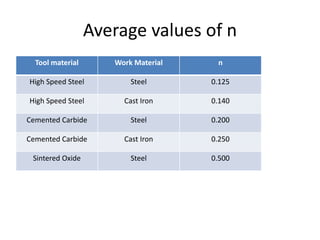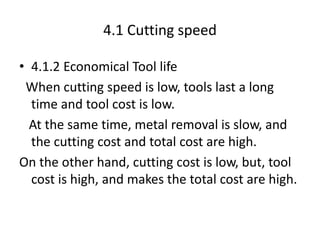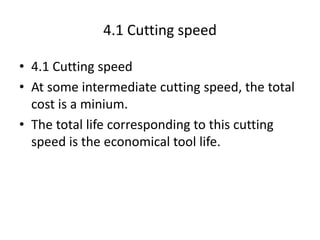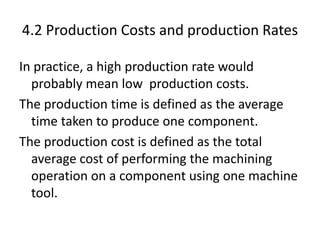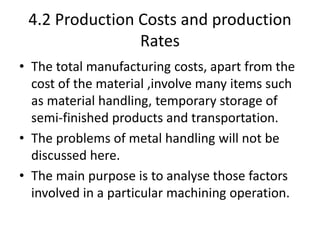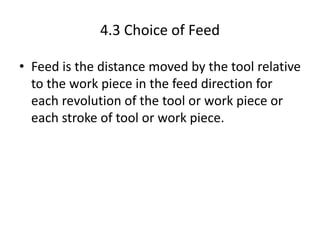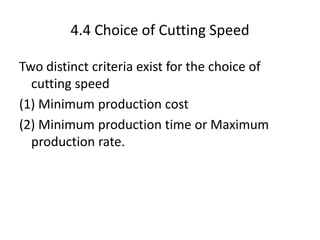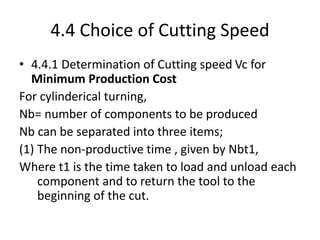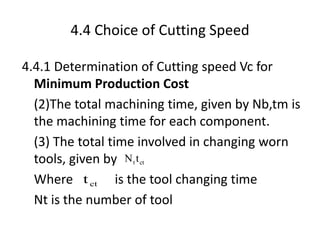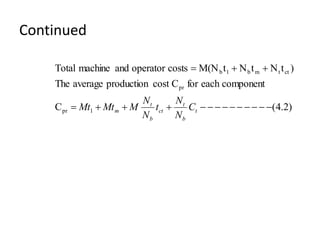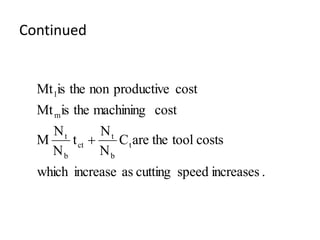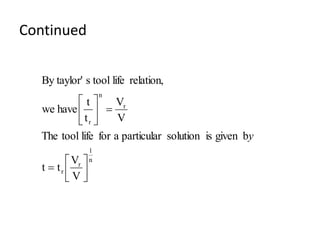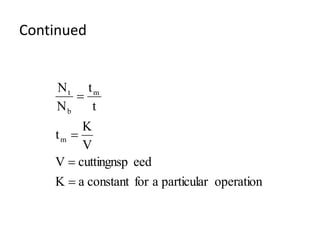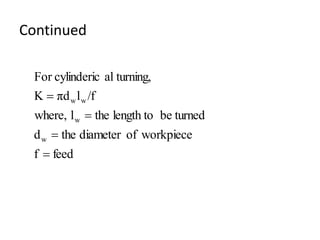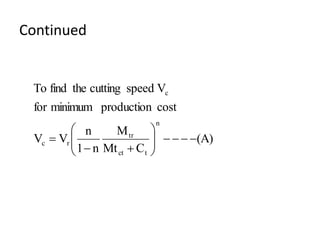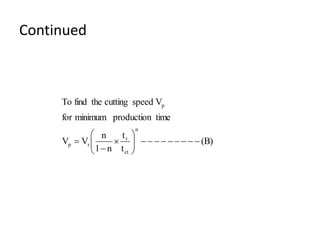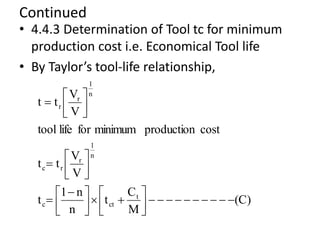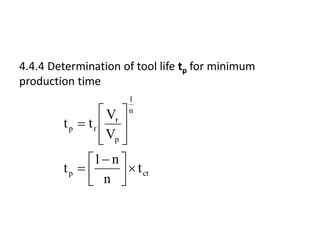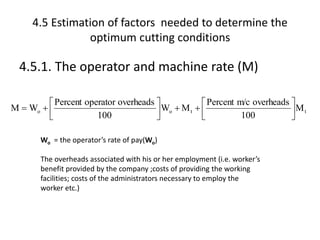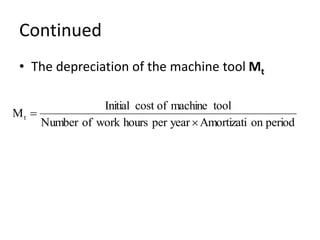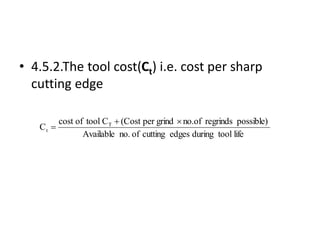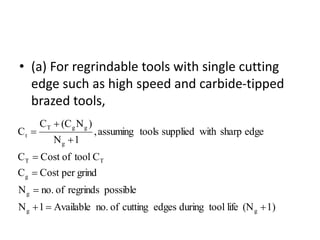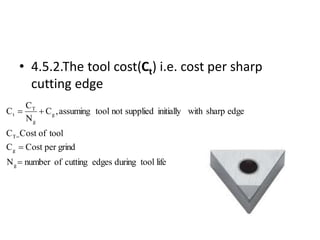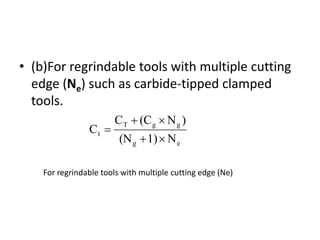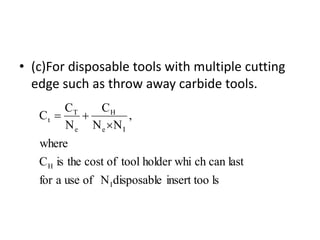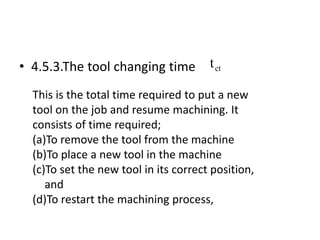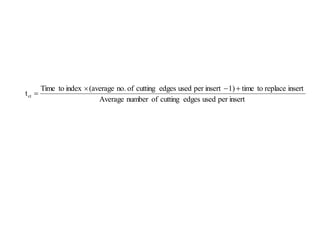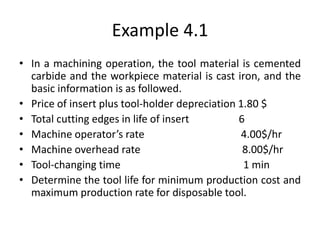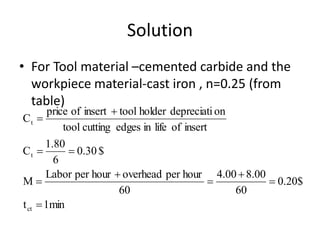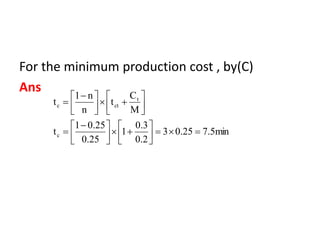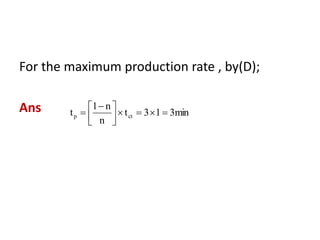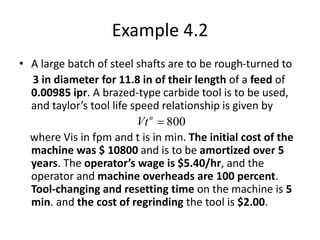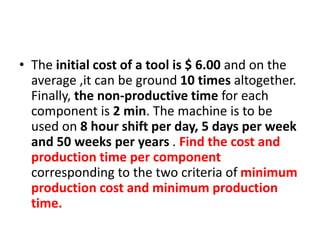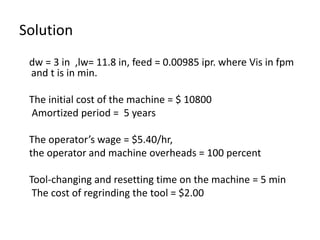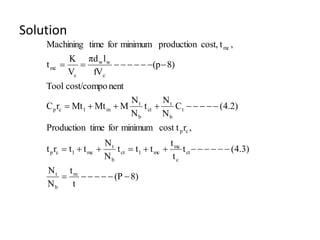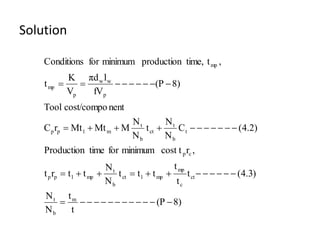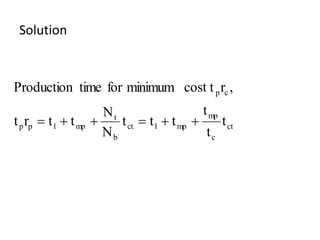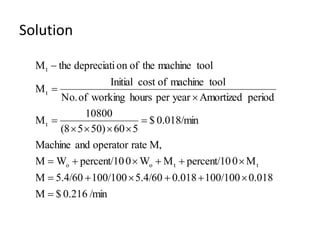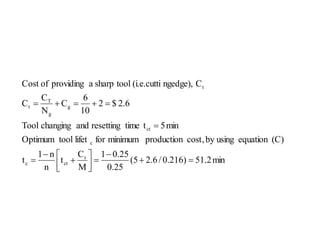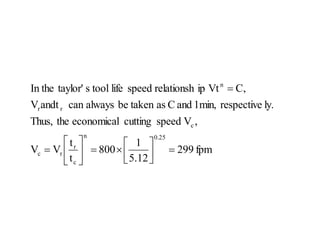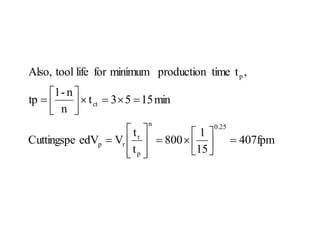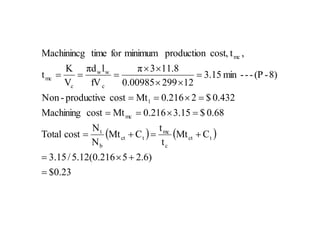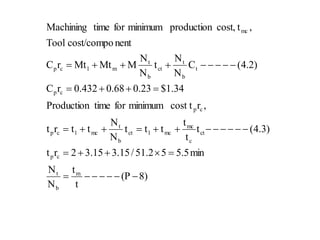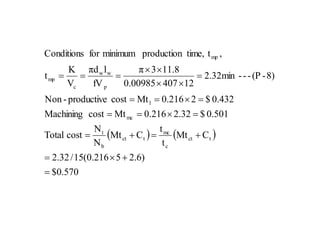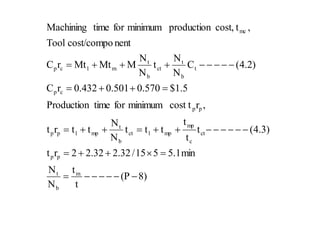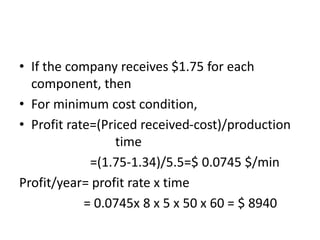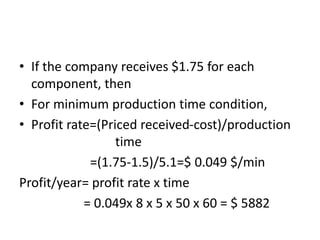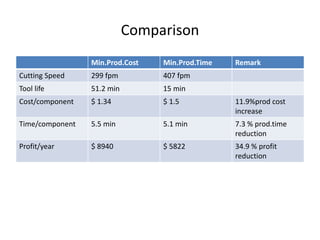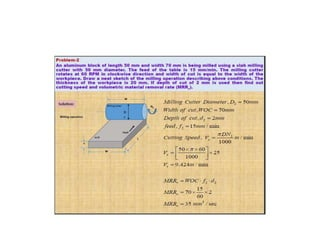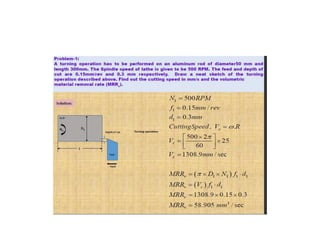1 sur 54
Publicité

### economics.pptx

1. Economics of metal cutting Dr. Cho Cho Myint Aye Mechanical Engineering Department Yangon Technological University
2. Course objectives -To know cutting speed, Tool life, cost per component, time per component for minimum production cost and min production time
3. 4.1 Cutting speed minute in life tool t fpm , speed cutting V -(4.1) - - - - - - - - - - - - - - - C Vt life tool and Speed 4.1.1 n   
4. Average values of n Tool material Work Material n High Speed Steel Steel 0.125 High Speed Steel Cast Iron 0.140 Cemented Carbide Steel 0.200 Cemented Carbide Cast Iron 0.250 Sintered Oxide Steel 0.500
5. 4.1 Cutting speed • 4.1.2 Economical Tool life When cutting speed is low, tools last a long time and tool cost is low. At the same time, metal removal is slow, and the cutting cost and total cost are high. On the other hand, cutting cost is low, but, tool cost is high, and makes the total cost are high.
6. 4.1 Cutting speed • 4.1 Cutting speed • At some intermediate cutting speed, the total cost is a minium. • The total life corresponding to this cutting speed is the economical tool life.
7. 4.2 Production Costs and production Rates In practice, a high production rate would probably mean low production costs. The production time is defined as the average time taken to produce one component. The production cost is defined as the total average cost of performing the machining operation on a component using one machine tool.
8. 4.2 Production Costs and production Rates • The total manufacturing costs, apart from the cost of the material ,involve many items such as material handling, temporary storage of semi-finished products and transportation. • The problems of metal handling will not be discussed here. • The main purpose is to analyse those factors involved in a particular machining operation.
9. 4.3 Choice of Feed • Feed is the distance moved by the tool relative to the work piece in the feed direction for each revolution of the tool or work piece or each stroke of tool or work piece.
10. 4.4 Choice of Cutting Speed Two distinct criteria exist for the choice of cutting speed (1) Minimum production cost (2) Minimum production time or Maximum production rate.
11. 4.4 Choice of Cutting Speed • 4.4.1 Determination of Cutting speed Vc for Minimum Production Cost For cylinderical turning, Nb= number of components to be produced Nb can be separated into three items; (1) The non-productive time , given by Nbt1, Where t1 is the time taken to load and unload each component and to return the tool to the beginning of the cut.
12. 4.4 Choice of Cutting Speed 4.4.1 Determination of Cutting speed Vc for Minimum Production Cost (2)The total machining time, given by Nb,tm is the machining time for each component. (3) The total time involved in changing worn tools, given by Where is the tool changing time Nt is the number of tool ct t t N ct t
13. Continued ) 2 . 4 ( C component each for C cost production average The ) t N t N t M(N costs operator and machine Total 1 pr pr ct t m b 1 b                  t b t ct b t m C N N t N N M Mt Mt
14. Continued . increases speed cutting as increase which costs tool the are C N N t N N M cost machining the is Mt cost productive non the is Mt t b t ct b t m 1 
15. Continued n 1 r r r n r V V t t b given is solution particular a for life tool The V V t t have we relation, life tool s By taylor'               y
16. Continued operation particular a for constant a K eed cuttingnsp V V K t t t N N m m b t    
17. Continued feed f workpiece of diameter the d turned be length to the l where, /f l πd K turning, al cylinderic For w w w w    
18. Continued (A) C Mt M n 1 n V V cost production minimum for V speed cutting the find To n t ct tr r c c               
19. Continued (B) t t n 1 n V V time production minimum for V speed cutting the find To n ct r r p p                    
20. Continued • 4.4.3 Determination of Tool tc for minimum production cost i.e. Economical Tool life • By Taylor’s tool-life relationship, (C) M C t n n 1 t V V t t cost production minimum for life tool V V t t t ct c n 1 r r c n 1 r r                                        
21. 4.4.4 Determination of tool life tp for minimum production time ct p n 1 p r r p t n n 1 t V V t t                  
22. 4.5 Estimation of factors needed to determine the optimum cutting conditions 4.5.1. The operator and machine rate (M) t t o o M 100 overheads m/c Percent M W 100 overheads operator Percent W M                 Wo = the operator’s rate of pay(Wo) The overheads associated with his or her employment (i.e. worker’s benefit provided by the company ;costs of providing the working facilities; costs of the administrators necessary to employ the worker etc.)
23. Continued • The depreciation of the machine tool Mt period on Amortizati year per hours work of Number tool machine of cost Initial Mt  
24. • 4.5.2.The tool cost(Ct) i.e. cost per sharp cutting edge life tool during edges cutting of no. Available possible) regrinds no.of grind per (Cost C tool of cost C T t   
25. • (a) For regrindable tools with single cutting edge such as high speed and carbide-tipped brazed tools, 1) (N life tool during edges cutting of no. Available 1 N possible regrinds of no. N grind per Cost C C tool of Cost C edge sharp with supplied tools assuming , 1 N ) N (C C C g g g g T T g g g T t         
26. • 4.5.2.The tool cost(Ct) i.e. cost per sharp cutting edge life tool during edges cutting of number N grind per Cost C tool of Cost C edge sharp with initially supplied not tool assuming , C N C C g g T g g T t     
27. • (b)For regrindable tools with multiple cutting edge (Ne) such as carbide-tipped clamped tools. e g g g T t N 1) (N ) N (C C C      For regrindable tools with multiple cutting edge (Ne)
28. • (c)For disposable tools with multiple cutting edge such as throw away carbide tools. ls insert too disposable N of use a for last can ch holder whi tool of cost the is C where , N N C N C C I H I e H e T t   
29. • 4.5.3.The tool changing time ct t This is the total time required to put a new tool on the job and resume machining. It consists of time required; (a)To remove the tool from the machine (b)To place a new tool in the machine (c)To set the new tool in its correct position, and (d)To restart the machining process,
30. insert per used edges cutting of number Average insert replace to time 1) insert per used edges cutting of no. (average index to Time tct    
31. Example 4.1 • In a machining operation, the tool material is cemented carbide and the workpiece material is cast iron, and the basic information is as followed. • Price of insert plus tool-holder depreciation 1.80 \$ • Total cutting edges in life of insert 6 • Machine operator’s rate 4.00\$/hr • Machine overhead rate 8.00\$/hr • Tool-changing time 1 min • Determine the tool life for minimum production cost and maximum production rate for disposable tool.
32. Solution • For Tool material –cemented carbide and the workpiece material-cast iron , n=0.25 (from table) 1min t \$ 20 . 0 60 00 . 8 00 . 4 60 hour per overhead hour per Labor M \$ 0.30 6 1.80 C insert of life in edges cutting tool on depreciati holder tool insert of price C ct t t          
33. For the minimum production cost , by(C) Ans 7.5min 0.25 3 0.2 0.3 1 0.25 0.25 1 t M C t n n 1 t c t ct c                                   
34. For the maximum production rate , by(D); Ans 3min 1 3 t n n 1 t ct p            
35. Example 4.2 • A large batch of steel shafts are to be rough-turned to 3 in diameter for 11.8 in of their length of a feed of 0.00985 ipr. A brazed-type carbide tool is to be used, and taylor’s tool life speed relationship is given by where Vis in fpm and t is in min. The initial cost of the machine was \$ 10800 and is to be amortized over 5 years. The operator’s wage is \$5.40/hr, and the operator and machine overheads are 100 percent. Tool-changing and resetting time on the machine is 5 min. and the cost of regrinding the tool is \$2.00. 800  n Vt
36. • The initial cost of a tool is \$ 6.00 and on the average ,it can be ground 10 times altogether. Finally, the non-productive time for each component is 2 min. The machine is to be used on 8 hour shift per day, 5 days per week and 50 weeks per years . Find the cost and production time per component corresponding to the two criteria of minimum production cost and minimum production time.
37. Solution dw = 3 in ,lw= 11.8 in, feed = 0.00985 ipr. where Vis in fpm and t is in min. The initial cost of the machine = \$ 10800 Amortized period = 5 years The operator’s wage = \$5.40/hr, the operator and machine overheads = 100 percent Tool-changing and resetting time on the machine = 5 min The cost of regrinding the tool = \$2.00
38. Solution The initial cost of a tool = \$ 6.00 Ng =10 times the non-productive time for each component ,t1= 2 min. The machine is to be used on 8 hour shift per day, 5 days per week and 50 weeks per years . To find the cost and production time per component corresponding to the two criteria of minimum production cost and minimum production time
39. Solution 8) (P t t N N .3) 4 ( t t t t t t N N t t r t , r cost t minimum for time Production ) 2 . 4 ( C N N t N N M Mt Mt r C nent cost/compo Tool 8) (p fV l πd V K t , t cost, production minimum for time Machining m b t ct c mc mc 1 ct b t mc 1 c p c p t b t ct b t m 1 c p c w w c mc mc                                     
40. Solution 8) (P t t N N ) 3 . 4 ( t t t t t t N N t t r t , r cost t minimum for time Production ) 2 . 4 ( C N N t N N M Mt Mt r C nent cost/compo Tool 8) (P fV l πd V K t , t time, production minimum for Conditions m b t ct c mp mp 1 ct b t mp 1 p p c p t b t ct b t m 1 p p p w w p mp mp                                             
41. Solution ct c mp mp 1 ct b t mp 1 p p c p t t t t t t N N t t r t , r cost t minimum for time Production      
42. Solution /min 0.216 \$ M 0.018 100/100 0.018 5.4/60 100/100 5.4/60 M M 0 percent/10 M W 0 percent/10 W M M, rate operator and Machine 0.018/min \$ 5 60 50) 5 (8 10800 M period Amortized year per hours working of No. tool machine of cost Initial M tool machine the of on depreciati the M t t o o t t t                      
43. min 2 . 51 ) 216 . 0 / 6 . 2 5 ( 25 . 0 25 . 0 1 M C t n n 1 t (C) equation using by , cost production minimum for lifet tool Optimum min 5 t time resetting and changing Tool 2.6 \$ 2 10 6 C N C C C ngedge), (i.e.cutti tool sharp a providing of Cost t ct c c ct g g T t t                   
44. fpm 299 5.12 1 800 t t V V , V speed cutting economical the Thus, ly. respective 1min, and C as taken be always can andt V C, Vt ip relationsh speed life tool s taylor' In the 0.25 n c r r c c r r n                 
45. 407fpm 15 1 800 t t V edV Cuttingspe min 15 5 3 t n n - 1 tp , t time production minimum for life tool Also, 0.25 n p r r p ct p                             
46.     23 . 0 \$ ) 6 . 2 5 216 . 0 ( 12 . 5 / 15 . 3 C Mt t t C Mt N N cost Total 0.68 \$ 3.15 0.216 Mt cost Machining 0.432 \$ 2 0.216 Mt cost productive - Non 8) - (P - - - min 3.15 12 299 0.00985 11.8 3 π fV l πd V K t , t cost, production minimum for time Machinincg t ct c mc t ct b t mc 1 c w w c mc mc                        
47. 8) (P t t N N min 5 . 5 5 2 . 51 / 15 . 3 15 . 3 2 r t .3) 4 ( t t t t t t N N t t r t , r cost t minimum for time Production 34 . 1 \$ 23 . 0 68 . 0 432 . 0 r C ) 2 . 4 ( C N N t N N M Mt Mt r C nent cost/compo Tool , t cost, production minimum for time Machining m b t c p ct c mc mc 1 ct b t mc 1 c p c p c p t b t ct b t m 1 c p mc                                     
48.     570 . 0 \$ ) 6 . 2 5 216 . 0 ( 15 / 32 . 2 C Mt t t C Mt N N cost Total 0.501 \$ 32 . 2 0.216 Mt cost Machining 0.432 \$ 2 0.216 Mt cost productive - Non 8) - (P - - - min 32 . 2 12 407 0.00985 11.8 3 π fV l πd V K t , t time, production minimum for Conditions t ct c mc t ct b t mc 1 p w w c mp mp                        
49. 8) (P t t N N min 1 . 5 5 15 / 32 . 2 32 . 2 2 r t .3) 4 ( t t t t t t N N t t r t , r cost t minimum for time Production 5 . 1 \$ 570 . 0 501 . 0 432 . 0 r C ) 2 . 4 ( C N N t N N M Mt Mt r C nent cost/compo Tool , t cost, production minimum for time Machining m b t p p ct c mp mp 1 ct b t mp 1 p p p p c p t b t ct b t m 1 c p mc                                     
50. • If the company receives \$1.75 for each component, then • For minimum cost condition, • Profit rate=(Priced received-cost)/production time =(1.75-1.34)/5.5=\$ 0.0745 \$/min Profit/year= profit rate x time = 0.0745x 8 x 5 x 50 x 60 = \$ 8940
51. • If the company receives \$1.75 for each component, then • For minimum production time condition, • Profit rate=(Priced received-cost)/production time =(1.75-1.5)/5.1=\$ 0.049 \$/min Profit/year= profit rate x time = 0.049x 8 x 5 x 50 x 60 = \$ 5882
52. Comparison Min.Prod.Cost Min.Prod.Time Remark Cutting Speed 299 fpm 407 fpm Tool life 51.2 min 15 min Cost/component \$ 1.34 \$ 1.5 11.9%prod cost increase Time/component 5.5 min 5.1 min 7.3 % prod.time reduction Profit/year \$ 8940 \$ 5822 34.9 % profit reduction
Publicité